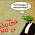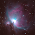This blog is for nonprofit, educational purposes - media is incorporated for educational purposes as outlined in § 107 of the U.S. Copyright Act.

## Wednesday, June 7, 2017

### Solar Eclipse Calculation Basics: Umbral Size

Certain Flat Earthers have complained that the Sun/Earth/Moon shadow diagrams aren't to scale properly and since I don't want to post a 10 GB image large enough to show anything useful to scale, I'm going to calculate it.

I'm going to calculate the Umbral size for a SOLAR eclipse.  This is where the Moon is a New Moon and it falls in between the Earth and the Sun and the SHADOW OF THE MOON falls on the Earth.  Not EVERY New Moon does this because the Moon's orbit is slightly tilted and an eclipse only happens with the Moon is at (or very near) the Node where the lunar orbit and ecliptic cross.

First the BASIC data we're going to focus on, using the average solar and lunar sizes and distances:

Earth - radius: 6378 km

Sun - radius / diameter: 695,700 km / 1,391,400 km
Sun - distance: 149,600,000 km (varies with time of year)

Moon - radius / diameter: 1737.5 km / 3,474 km
Moon - distance: 384,400 km (varies with time of month)
Moon - surface distance: 384,400 km - (Earth|radius) = 378,022 km

So I just fired up Celestia and used the Navigator option to find the next eclipse and then I moved *MY VIEW* so I could see the Moon and the eclipse shadow on the Earth.  Moving *MY VIEW* doesn't change the relationship between the Sun/Moon/Earth so the shadow doesn't move when *MY VIEW* moves.  Easy as π

If I move my view down to the middle of the dark spot and we look back towards the Moon/Sun, we see the eclipse:

Ok -- so from here the Moon and Sun are lined up.  Now imagine we're looking DEAD CENTER of the moon and just consider the top half of that picture...

We can find the SLOPE of a line from here to Moon very easily.

SLOPE is Rise/Run -- remember that from elementary school?

Rise = Moon Radius (1,737.5 km)
Run = Distance To Moon (378,022 km)

SLOPE = 1737.5/378022

That's the Slope of that line, we can convert a slope to an angle using arctan(SLOPE).

arctan(1737.5/378022) in arc minutes ~ 15.8'

Now that's just half of the moon -- double that to get the full angular size of the moon or about 31' arc minutes.  This matches to reality, although the EXACT angular size of the moon varies by about 14% as it orbits the Earth because it's distance is changing through the month.Image Credit

This is, BTW, also the "Law of Perspective".

Now do the same thing for the Sun:

Sun - radius / diameter: 695,700 km / 1,391,400 km
Sun - distance 149,600,000 km

arctan(695700/149600000) in arc minutes ~ 15.99'

So we can see the Moon and Sun should appear almost the same size with the Sun just a hair larger using these values.

But at this distance the rays from the TOP part of the Sun and the BOTTOM part of the Sun are going to have a SLIGHT angle.  The Sun's rays are almost parallel ONLY because of the great distances involved but there is still a SLIGHT angle.TINY IMAGE, Click to Enlarge

So now let's go the other direction and take the angle from the the top of the Sun to the top of the Moon and we can see how far out into space that angle would cross with the one coming from the bottom.

This time our Rise is shorter by the lunar radius and our Run is shorter by the lunar distance.

arctan((695700-1737.5)/(149600000-378022)) in degrees ~ 0.2665°

To extend this angle towards the Earth we need to find the length of the adjacent side since the opposite side here is 1 Moon radius:

We know the trigonometric relationship is:

tan(A) = opposite / adjacent

And we already determined our angle is

A = arctan((695700-1737.5)/(149600000-378022))

But the tan(arctan()) term cancels out, leaving us with a very simple math problem:

((695700-1737.5)/(149600000-378022)) = 1737.5 / adjacent

Or approximately 373613 km

Of course, the exact distances from Earth-to-Moon and Earth-to-Sun vary so the size and length of this shadow vary accordingly.

If the distance of the Umbra is shorter than the distance to the Earth, as we calculated here, then we actually see the Antumbra and you see the Ring Of Fire, or annulus, of the Sun and this would be called an Annular Eclipse.

If the moon is a bit closer than the above 'average' value we used then it has a larger angular size and we get a 'normal' Umbral eclipse.  The lunar distance varies from around 357,208 km to 406,603 km -- and the exact distance at Perigee and Apogee vary because of the influence of the Sun (and a few smaller influences).

So, not only does this exactly match what we observe it explains why AND PREDICTS WHEN you can expect to see each type of eclipse.

To calculate the SIZE of the Umbral/Antumbral shadow we would simply take the half-angle and project it out the difference in the distance between the the length of the shadow and the distance to the Earth -- and then double that (because we have a half-angle)...

I'll do it step-by-step first, following the same model as above, but this time we will take the lunar distance as 360,000 km which is 353,622 km from the Earth's surface.

Our first equation to find the Umbral length is:

((695700-1737.5)/(149600000-353622)) = 1737.5 / adjacent

So we get an Umbral length of approximately 373674 km which is greater than the distance to the Earth, 353,622 km this time.

Now we need to take the Umbral length and find where it intersects the Earth's surface, so that's our Umbral length minus the distance to the Moon from the Earth's surface (353,622 km in this case).

And 373674-353622 gives us our intersection at 20052 km - so we take our angle out with the same formula as before:
tan(A) = opposite / adjacent

Except now our adjacent is 20052 and we solve for opposite:

((695700-1737.5)/(149600000-353622)) = opposite / 20052 ~ 90.2373 km

And we double that to get the total width of our Umbra: ~ 127 km

[NOTE: because the Earth surface is actually curved and bumpy the actual width varies slightly from this idealized approximation, but only very slightly. To solve it fully you need to find the surface of intersection between the shadow cone and the exact bumpy shape of the Earth (or at least the Ellipsoid approximation]

We can also simplify this down to the following (just make SURE all your units are the same, you get out whatever you put in -- miles, km, whatever).

m = moon radius
M = moon distance (from Earth's surface)
s = sun radius
S = sun distance

Umbral Diameter ~ 2(M s - m S)/(M - S) where m=1737.5,M=353622,s=695700,S=149600000 ~ 186.473 km

where m=1737.5,M=360000,s=695700,S=149600000,E=6378
where m=1738,M=(356400-6378),s=696000,S=152100000

Can you find the distance from the Earth to the Moon is required to exactly match the Sun's size (for a given solar distance)?

HINT: You want their slopes to match.

Select the below text to see the answer.

1737.5/X = 695700/149600000 ~ 373624 km

How big is the Umbra at that point?

The Earth to Sun distance also changes with the years, Astropixels has a nice chart.

If you want to go out and take accurate measurements you need to find the CURRENT distance to the Moon and to the Sun at YOUR actual location.

Can you calculate the Penumbra now?  How would you go about that?

It's a little more complex but the principles are the same.  Left as an exercise to the reader.

## Math Summary

m = moon radius
M = moon distance (from Earth's surface)
s = sun radius
S = sun distance (from Earth's surface)

θ = arctan((s-m)/(S-M)) -- the angle of the shadow
adjacent = opposite / tan(θ) -- trigonometric identity, to find shadow length
tan(arctan()) cancels out, and opposite is moon radius
adjacent = m / ((s-m)/(S-M))

approx Umbral Diameter ~ 2 * (adjacent - M) * tan(θ)
substitute
approx Umbral Diameter ~ 2 * ((m / ((s-m)/(S-M))) - M) * ((s-m)/(S-M))
simplify
approx Umbral Diameter ~ 2 * (m S - M s) / (S - M)

TECHNICAL NOTE: the "moon radius" here should actually be the lunar horizon radius because the light is tangent off the sphere of the Moon.  For this you can use θ = arcsin((s-m)/(S-M)).  However, at these distances it makes little difference and gives us a simple algebraic way to approximate the size of the umbra.

1.1.